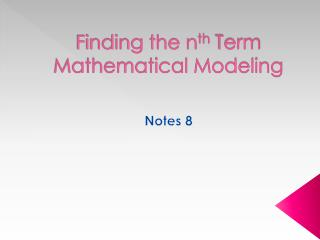DownloadDownload PresentationFinding the n th Term Mathematical Modeling

# Finding the n th Term Mathematical Modeling

Download Presentation## Finding the n th Term Mathematical Modeling

- - - - - - - - - - - - - - - - - - - - - - - - - - - E N D - - - - - - - - - - - - - - - - - - - - - - - - - - -
##### Presentation Transcript

1. Essential Learnings • Students will understand and be able to determine the function rule for a sequence. • Students will understand and be able to model mathematical problems.

2. Vocabulary • Linear function – a function that creates a line when graphed. • Function rule – a rule that gives you the nth term in a sequence.

3. Example 1 Tell whether or not the rule is a linear function.

4. Example 2 Tell whether or not the rule is a linear function.

5. Example 3 Find the function rule for each sequence. Then find the 50th term in the sequence.

6. Example 4 Find the function rule for each sequence. Then find the 50th term in the sequence.

7. Example 5 Find the rule of the number of tiles in the nth figure. Then find the number of tiles in the 100th figure.

8. Mathematical Modeling

9. Handshake Problem

10. Rectangular Numbers Rectangular numbers are numbers that can be arranged in a rectangular pattern of dots.

11. Triangular Numbers Triangular numbers are numbers that can be arranged in a triangular pattern of dots.

12. Assignment nth Term Worksheet Mathematical Modeling WS Quiz - Monday Unit 1 Test – Friday (9/23)